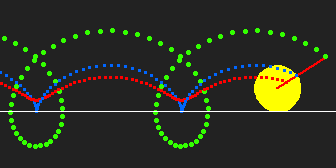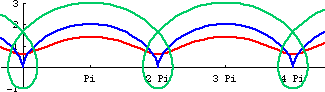# TrochoidBoundary formed by trochoids of different parameters.

## Description

Trochoid describe a family of curves. (See: Curve Family Index) Trochoid is defined as the trace of a point fixed on a circle that rolls along a line. Sometimes the name trochoid is used to mean hypotrochoid and epitrochoid. (curve traced by rolling circle on another circle) More generally, trochoid is any curve that is the locus of a point fixed to a curve A, while A rolls on another curve B without slipping.Trochoid Tracing. r:=1, h:={.5, 1, 2.5}.

## Formula

Let the radius of the rolling circle be r and the distance from the tracing point Q to the center of the circle be h.

• Parametric: {r t - h Sin[t], r - h Cos[t]}. Periodicy: 2 π.

## Properties

### Special Cases

• If Q lies on the rolling circle, it is a cycloid.
• If Q lies outside the rolling circle, it is sometimes called prolate (extended) cycloid.
• If Q lies inside the rolling circle, it is curtate (contracted) cycloid.Cyloid (blue), extended cycloid (green), contracted cycloid (red).This animation shows the trochoid with parameters r:=1 and h increase from 0 to 3. The radial of the curve is also ploted as black dots. When r==h, it is a cycloid and its radial is a circle.

## Related Web Sites

Robert Yates: Curves and Their Properties.

If you have a question, put \$5 at patreon and message me.